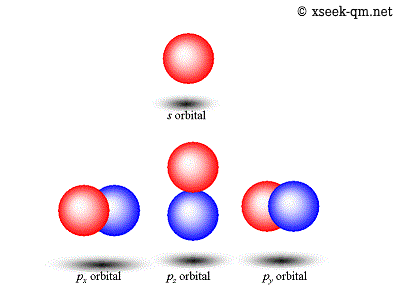# The wave function of quantum mechanics

2013/6/30
Published 2012/11/4

I would like to consider the wave function of the electron in a hydrogen atom, in this page.The wave function of quantum mechanics

## Rotation axis of spherical harmonics of atomic orbital

According to the atomic model of Bohr, an electron is rotating around the nucleus. We express the atomic orbital by using spherical harmonics. However, we are not able to find a revolution in the following figure of the atomic orbital.We see the shape that consists of a red ball and a blue ball of the second line in the above figure. The shape of the second line must express the rotation of the electron because it has angular momentum. However, we are not able to find any rotation axis.

There is a following way to express the wave function.

1. We represent the amplitude as the brightness of the drawing.
2. We represent the phase as hue.

We express the animation of electron's rotation around the nucleus by using this way.

I thought that I express the animation by using JavaScript. I will show you in the next section that animation.

## Animation of the rotation of the electron

We watch the GIF animation of the wave function of electron rotating on 4f orbital of hydrogen atom in the following page.JavaScript animation of the wave function of electron

([State] = (431), [Number] = 51200)

I also uploaded the animation to youtube.

I am making the movie with the following steps.

(1) To place the point in proportion to the observation probability, by Monte Carlo method.
(2) To give color to the point based on the phase.
(3) To change the phase of each point per time.

Then, we see the rotation only by changing the phase.

Animation of wave function of an electron rotating in the hydrogen atom is as follows.JavaScript animation of the wave function of electron

([State] = (431), [Number] = 51200)

### State

The initial state is the state (n, l, m) = (4, 3, 1). (n: the principal quantum number, l: the azimuthal quantum number, m: the magnetic quantum number)

If you press the button "State," you can choose one of the states, (1, 0, 0), (2, 1, 1), (3, 2, 1), and (3, 2, 2). The state (n, l, m) = (1, 0, 0) does not rotate. The phase changes in the whole.

### Number of points

Initial number of points is 51200. If you do not have an available capacity to machine power, please consider decreasing the number of points by pressing the button "Number."

## Commentary

I order to express the animation, I used Canvas by JavaScript. It works on the following browsers.

• IE 6 or later
• Google Chrome 4 or later
• Firefox 1.5 or later
• Safari for Windows 1.3 or later
• Opera 9 or later
• Safari for iOS 4 or later (iPhone)

For IE6, IE7 and IE8 those do not support Canvas, I used JavaScript library "uuCanvas.js" that offers HTML5::Canvas compatible function.

Related Articles: